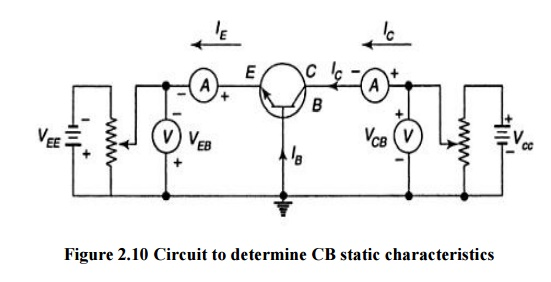Home | | Electronic Devices and Circuits | Common base(CB) configuration of Transistor

# Common base(CB) configuration of Transistor

In common base configuration circuit is shown in figure. Here base is grounded and it is used as the common terminal for both input and output.

COMMON BASE(CB) CONFIGURATION

In common base configuration circuit is shown in figure. Here base is grounded and it is used as the common terminal for both input and output.It is also called as grounded base configuration. Emitter is used as a input terminal where as collector is the output terminal.

## Input characteristics:

It is defined as the characteristic curve drawn between input voltage to input current whereas output voltage is constant.

To determine input characteristics, the collector base voltage VCB is kept constant at zero and emitter current IE is increased from zero by increasing VEB.This is repeated for higher fixed values of VCB.

A curve is drawn between emitter current and emitter base voltage at constant collector base voltage is shown in figure 2.11.When VCB is zero EB junctions is forward biased. So it behaves as a diode so that emitter current increases rapidly.## Ø          Output Characteristics

It is defined as the characteristic curve drawn between output voltage to output current whereas input current is constant.To determine output characteristics, the emitter current IE is kept constant at zero and collector current Ic is increased from zero by increasing VCB.This is repeated for higher fixed values of IE.

From the characteristic it is seen that for a constant value of IE, Ic is independent of VCB and the curves are parallel to the axis of VCB.As the emitter base junction is forward biased the majority carriers that is electrons from the emitter region are injected into the base region.

In CB configuration a variation of the base-collector voltage results in a variation of the quasi- neutral width in the base. The gradient of the minority-carrier density in the base therefore changes, yielding an increased collector current as the collector-base current is increased. This effect is referred to as the Early effect.A comparison of CB, CE and CC ConfigurationsStudy Material, Lecturing Notes, Assignment, Reference, Wiki description explanation, brief detail
Electronic Devices and Circuits : Transistors : Common base(CB) configuration of Transistor |